# Digital PLL's -- Part 2

In Part 1, we found the time response of a 2nd order PLL with a proportional + integral (lead-lag) loop filter.  Now let’s look at this PLL in the Z-domain [1, 2].  We will find that the response is characterized by a loop natural frequency ωn and damping coefficient ζ.

Having a Z-domain model of the DPLL will allow us to do three things:

1. Compute the values of loop filter proportional gain KL and integrator gain KI that give the desired loop natural frequency and damping.  Deriving these formulae is somewhat involved, but the good news is we only have to do it once.
2. Compute the linear-system time response to a step in the reference phase.
3. Compute the frequency response.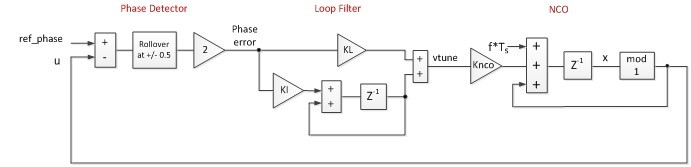Figure 1.  DPLL Time Domain Model Block Diagram

Figure 1 is the time-domain DPLL model we derived in part 1.  To convert this to a useful Z-domain model, we replace the accumulators in the Loop Filter and NCO with the transfer function  z-1/(1 – z-1), who’s numerator and denominator we can multiply by z to get 1/(z – 1).  This gives us the model in Figure 2, where we have also indicated the phase detector gain Kp.Figure 2.  DPLL in the Z-domain

The open-loop response of this system is the product of the transfer functions of the three blocks:

$$G_1(z) = \frac{K_pK_LK_{nco}}{z-1} + \frac{K_pK_IK_{nco}}{(z-1)^2} \tag{1}$$

### 2nd Order System in s and z

A 2nd order continuous-time system with a Lead-lag filter is shown in Figure 3. [3, 4].  See Appendix B for a derivation of the closed-loop response.  If we convert this to the Z-domain, we’ll see the response has the same form as that of our DPLL.  This will allow us to relate KL and KI of the DPLL to ωn and ζ.Figure 3.  2nd order system in s with a zero in the closed-loop response

To convert this system to the z-domain equivalent, make the following replacement:

$$s \rightarrow \frac{z-1}{T_s}$$

This approximation is valid as long as the loop natural frequency is much less than the sample frequency (see Appendix C).  The Z-domain block diagram is shown in Figure 4, where we have allowed for the possibility that the loop filter could have a sample rate Ts_filt  different from the NCO sample rate Ts_nco.Figure 4.  2nd order system in the z-domain

The open-loop response G2(z)  is:

$$G_2(z) = \frac{2\zeta\omega_nT_{s\_nco}}{z-1} + \frac{T_{s\_filt}T_{s\_nco}\omega_n^2}{(z-1)^2} \tag{2}$$

By equating open-loop response G1 of our DPLL to G2, we can find KL and KI in terms of ωn and ζ.

Equating G1 and G2:

$$\frac{K_pK_LK_{nco}}{z-1} + \frac{K_pK_IK_{nco}}{(z-1)^2} = \frac{2\zeta\omega_nT_{s\_nco}}{z-1} + \frac{T_{s\_filt}T_{s\_nco}\omega_n^2}{(z-1)^2}$$

Solve for KL and KI:

$K_pK_LK_{nco} = 2\zeta\omega_nT_{s\_nco}$        →        $K_L = 2\zeta\omega_n/K_p\; * \; T_{s\_nco}/K_{nco}$          (3)

$K_pK_IK_{nco} = T_{s\_filt}T_{s\_nco}\omega_n^2$      →     $K_I = T_{s\_filt}\omega_n^2/K_p \; * \; T_{s\_nco}/K_{nco}$          (4)

A given DPLL design has defined values for Ts_filt,Ts_nco, Kp, and Knco.  Given those values, KL and KI are uniquely determined by the choice of ωn and ζ.    Note that the units of Kp are cycle-1.  See Appendix D for an alternate form of the equations for KL and KI.

### Computing the Closed-loop response

The closed loop phase response of the DPLL in Figure 2 is given by:

$$CL(z) = \frac{Z[u]}{Z[ref\_phase]} = \frac{G_1}{1 + G_1}$$

Thus from equation 1,

$$\frac{K_pK_LK_{nco}} {z-1} + \frac{K_pK_IK_{nco}} {(z-1)^2}$$

$CL(z) =$                       __________________________________

$$1 + \frac{K_pK_LK_{nco}} {z-1} + \frac{K_pK_IK_{nco}} {(z-1)^2}$$

$$CL(z) = \frac {b_0 + b_1z^{-1}} {1 + a_1z^{-1} + a_2z^{-2}} \qquad (5)$$

Where

b0 = KpKLKnco
b1 = KpKIKnco - KpKLKnco
a1 = KpKLKnco – 2
a2 = 1 + KpKIKnco - KpKLKnco

Equation 5 is in the form of an IIR filter transfer function, which allows for straightforward computation of the time and frequency responses in Matlab.

Example

This example uses the same parameters as Example 2 in Part 1.  We will compute KL and KI, then we will find the time and frequency response using CL(z).  For this example, fs_nco = fs_filt = fs.  The Matlab script is listed in Appendix A.   The DPLL parameters are as follows.  As you can see, not all of the parameters from the time domain model apply to the Z-domain model.

fs                                                  25 MHz
Reference frequency                   NA
Initial reference phase                 NA
NCO initial frequency error          NA
Knco                                             1/4096
Kp                                                2 cycle-1
fn                                                 400 Hz   loop natural frequency.  fn = ωn/(2π)
ζ                                                  1.0           loop damping coefficient

1.  Find KL and KI

KL= 2*zeta*wn*Ts/(KP*Knco)      % loop filter proportional gain
KI= wn^2*Ts^2/(KP*Knco)         % loop filter integral gain
KL = 0.4118
KI = 2.0698e-005


2.  Compute the time response to a step in the reference phase.  Since CL(z) is in the form of a digital filter transfer function, we can find the time response using the Matlab "filter"  function.

b0= KP*KL*Knco;
b1= KP*Knco*(KI - KL);
a1= KP*KL*Knco - 2;
a2= 1 + KP*Knco*(KI - KL);
b= [b0 b1];         % numerator coeffs
a= [1 a1 a2];       % denominator coeffs
x= ones(1,N);       % step function
y= filter(b,a,x);   % step response
pe= y-1;            % phase error response


The phase error response is shown in Figure 5.  Because this model is linear, the non-linear acquisition behavior we saw in the time-domain model of Part 1 (Figure 3.4) is missing.  Thus we see that the Z-domain model is less capable than the time-domain model for computing the time response.  Finally, one detail worth mentioning:  the response has some overshoot.  This is caused by the zero in CL(z).  (An all-pole system would not have overshoot for ζ = 1).

3.  Compute the frequency response CL(z).

u = 0:.1:.9;
f= 10* 10 .^u;                  % log-scale frequencies
f = [f 10*f 100*f 1000*f];
z = exp(j*2*pi*f/fs);           % complex frequency z
CL= (b0 + b1*z.^-1)./(1 + a1*z.^-1 + a2*z.^-2);  % closed-loop response
CL_dB= 20*log10(abs(CL));
semilogx(f,CL_dB),grid


The closed-loop frequency response is shown in Figure 6.  Comparing this response to that of the equivalent continuous-time system in Figure B.2, we see that they match.  Note the peak in the response occurs near the loop natural frequency of 400 Hz.  The slope of the response in the stopband is -20 dB/decade.Figure 5.  Phase Error for unit-step change in reference phase.  fn = 400 Hz, ζ = 1.0.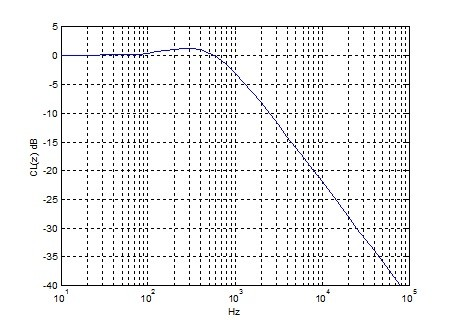Figure 6.  Closed-Loop Frequency Response.  fn = 400 Hz, ζ = 1.0.

4.  Plot the step response and the frequency response for different values of damping.  Figure 7 shows the step response and Figure 8 shows the frequency response.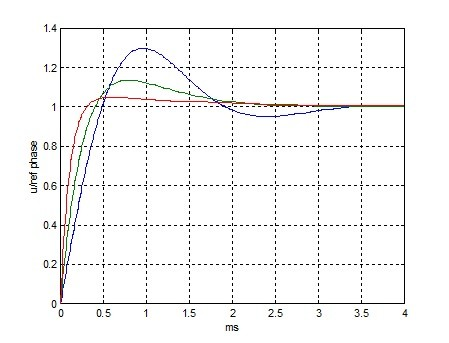Figure 7.  Step Response.  fn = 400 Hz;  ζ = 0.5 (blue), 1.0 (green), 2.0 (red)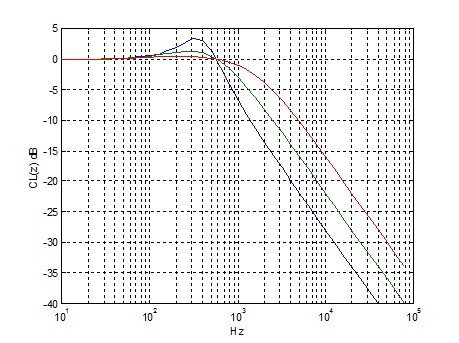Figure 8.  Closed-Loop Frequency Response.  fn = 400 Hz;  ζ = 0.5 (blue), 1.0 (green), 2.0 (red)

## Appendix A.  Z-Domain model of DPLL with fn = 400 Hz

%pll_response_z2.m     nr  5/24/16
% Digital 2nd order type 2 PLL
% step response and closed loop frequency response
Knco= 1/4096;       %   NCO gain
KP= 2;              % 1/cycles  phase detector gain
wn = 2*pi*400;      % rad/s   loop natural frequency
fs = 25e6;         % Hz sample rate
zeta = 1;           % damping factor
Ts= 1/fs;           % s  sample time
KL= 2*zeta*wn*Ts/(KP*Knco)      % loop filter proportional gain
KI= wn^2*Ts^2/(KP*Knco)         % loop filter integral gain
% Find coeffs of closed-loop transfer function of u/ref_phase
% CL(z) = (b0 + b1z^-1)/(a2Z^-2 + a1z^-1 + 1)
b0= KP*KL*Knco;
b1= KP*Knco*(KI - KL);
a1= KP*KL*Knco - 2;
a2= 1 + KP*Knco*(KI - KL);
b= [b0 b1];         % numerator coeffs
a= [1 a1 a2];       % denominator coeffs
% step response
N= 100000;
n= 1:N;
t= n*Ts;
x= ones(1,N);       % step function
y= filter(b,a,x);   % step response
pe = y – 1;         % phase error response
plot(t*1e3,pe),grid
xlabel('ms'),ylabel('Phase Error = u/ref-phase -1'),figure  %plot phase error
% Closed-loop frequency response
u = 0:.1:.9;
f= 10* 10 .^u;                  % log-scale frequencies
f = [f 10*f 100*f 1000*f];
z = exp(j*2*pi*f/fs);           % complex frequency z
CL= (b0 + b1*z.^-1)./(1 + a1*z.^-1 + a2*z.^-2);    % closed-loop response
CL_dB= 20*log10(abs(CL));
semilogx(f,CL_dB),grid
xlabel('Hz'),ylabel('CL(z) dB')


## Appendix B.  2nd order continuous-time system closed loop response in s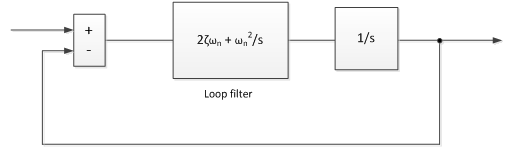Figure B.1.  2nd order system in s with a zero in the closed-loop response

$A(s) = 2\zeta\omega_n + \frac {\omega_n^2} {s}$        lead-lag filter

$G(s) = \frac {1} {s} A(s) = \frac {2\zeta\omega_n} {s} + \frac {\omega_n^2} {s^2}$

$CL(s) = G/(1+G)$

$CL(s) = \frac {2\zeta\omega_n + \omega_n^2} {s^2 + 2\zeta\omega_n + \omega_n^2}$

where

ωn= 2πfn = loop natural frequency
ζ= damping factorFigure B.2.  Closed-Loop Frequency Response.  fn = 400 Hz, ζ = 1.0.

## Appendix C.  Converting H(s) to H(z)

This is a way to approximate H(s) when a system’s passband frequency range is much less than the sample frequency.  We choose this method because it results in a block diagram and transfer function that have the same form as that of our DPLL in Figure 2.

The definition of z is:

z = exp(sTs)

where s is complex frequency and Ts is the sample time.  If we approximate z by the first two terms in the Taylor series for ex, we have

z ~= 1 + sTs                  (1)

Here we are assuming 2πfTs  << 1, or f/fs << 1/2π.  For our examples, we have been using fn = 5 kHz or less and fs = 25 MHz.  So fn/fs = .0002 << 1/2π.

Rearranging equation 1, we get

s ~= (z – 1)/Ts

To convert H(s) to H(z) we replace each occurrence of the variable s by (z – 1)/Ts

## Appendix D.  Alternative formulae for loop filter coefficients

The gain block in front of the NCO has gain Knco.  The output frequency of the NCO due just to Vtune is

f   = Vtune*Knco*fs_nco

If we define Kv = Knco*fs_nco        Hz,

then Knco can be replaced by Kv*Ts_nco in the formulae for KL and KI.  So equations 3 and 4 become:

$K_L = 2\zeta\omega_n/(K_pK_v)$

$K_I = \omega_n^2T_{s\_filt}/(K_pK_v)$

Here, the units of Kp are cycle-1, which is consistent with Kv in Hz.  Alternative units for Kp and Kv are radian-1 and rad/s, respectively.

## References

1.  Gardner, Floyd M., Phaselock Techniques, 3rd Ed., Wiley-Interscience, 2005, Chapter 4.

2.  Rice, Michael, Digital Communications, a Discrete-Time Approach, Pearson Prentice Hall, 2009, Appendix C.

3.  Gardner, Section 2.2.3.

4.  Rice, C.1.3

6/9/2016            Neil Robertson

[ - ]
Comment by November 10, 2019Hello,

I was wondering if this is right:

"To convert this to a useful Z-domain model, we replace the accumulators in the Loop Filter and NCO with the transfer function (z^-1)/(1 – z^-1)"

I've done this many times and I can only get the equation 1/(1 – z^-1). Where does the numerator z^-1 come from??

[ - ]
Comment by November 10, 2019Hi,

The numerator term z^-1 occurs if the delay element is in the forward path of the accumulators, as shown in Figure 1.  If the delay element is in the feedback path, the numerator term is 1, as you mention.

Note that if the NCO accumulator has no forward delay, there is no delay in the PLL loop at all, which can cause timing problems in the real world.

regards,

Neil

[ - ]
Comment by December 13, 2021Hi, Neil

Great thanks for your document. I am wondering whether the equation (5) should be something like below. Right?

CL(z)=(b_1 z^(-1)+b_2 z^(-2))/(1+a_1 z^(-1)+a_2 z^(-2) )      (5)

[ - ]
Comment by December 14, 2021Yarn,

You may be right. Your equation's numerator could be rewritten as:

num = z^-1(b1 + b2z^-1)

or, using conventional subscripts:

num= z^-1(b0 + b1z^-1)

Compared to equation 5, this has an extra zero at z = 0, which corresponds to a delay of one sample.  This would account for the unit delay in the NCO.

As a practical matter, I don't think it affects the response.

regards,

Neil

[ - ]
Comment by December 15, 2021Hi, Neil

Yes, thanks. It just addes a sample delay which will not impact the loop dynamics.

To post reply to a comment, click on the 'reply' button attached to each comment. To post a new comment (not a reply to a comment) check out the 'Write a Comment' tab at the top of the comments.